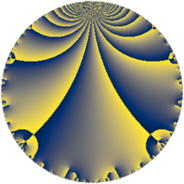# Properties

 Label 98.4.aLevel $98$ Weight $4$ Character orbit 98.a Rep. character $\chi_{98}(1,\cdot)$ Character field $\Q$ Dimension $10$ Newform subspaces $8$ Sturm bound $56$ Trace bound $3$

# Related objects

## Defining parameters

 Level: $$N$$ $$=$$ $$98 = 2 \cdot 7^{2}$$ Weight: $$k$$ $$=$$ $$4$$ Character orbit: $$[\chi]$$ $$=$$ 98.a (trivial) Character field: $$\Q$$ Newform subspaces: $$8$$ Sturm bound: $$56$$ Trace bound: $$3$$ Distinguishing $$T_p$$: $$3$$

## Dimensions

The following table gives the dimensions of various subspaces of $$M_{4}(\Gamma_0(98))$$.

Total New Old
Modular forms 50 10 40
Cusp forms 34 10 24
Eisenstein series 16 0 16

The following table gives the dimensions of the cuspidal new subspaces with specified eigenvalues for the Atkin-Lehner operators and the Fricke involution.

$$2$$$$7$$FrickeDim.
$$+$$$$+$$$$+$$$$3$$
$$+$$$$-$$$$-$$$$2$$
$$-$$$$+$$$$-$$$$1$$
$$-$$$$-$$$$+$$$$4$$
Plus space$$+$$$$7$$
Minus space$$-$$$$3$$

## Trace form

 $$10 q - 6 q^{3} + 40 q^{4} + 26 q^{5} + 20 q^{6} + 126 q^{9} + O(q^{10})$$ $$10 q - 6 q^{3} + 40 q^{4} + 26 q^{5} + 20 q^{6} + 126 q^{9} - 4 q^{10} - 12 q^{11} - 24 q^{12} - 74 q^{13} + 92 q^{15} + 160 q^{16} + 40 q^{17} + 128 q^{18} - 82 q^{19} + 104 q^{20} - 80 q^{22} + 268 q^{23} + 80 q^{24} + 310 q^{25} - 76 q^{26} - 180 q^{27} - 328 q^{29} - 432 q^{30} - 308 q^{31} + 320 q^{33} + 376 q^{34} + 504 q^{36} - 916 q^{37} + 156 q^{38} - 1352 q^{39} - 16 q^{40} - 288 q^{41} + 288 q^{43} - 48 q^{44} + 242 q^{45} - 80 q^{46} - 12 q^{47} - 96 q^{48} - 1192 q^{50} - 884 q^{51} - 296 q^{52} - 1152 q^{53} - 40 q^{54} + 184 q^{55} + 156 q^{57} + 24 q^{58} + 62 q^{59} + 368 q^{60} - 182 q^{61} - 328 q^{62} + 640 q^{64} + 476 q^{65} - 256 q^{66} + 3044 q^{67} + 160 q^{68} + 656 q^{69} - 704 q^{71} + 512 q^{72} + 612 q^{73} - 232 q^{74} - 530 q^{75} - 328 q^{76} + 128 q^{78} + 2028 q^{79} + 416 q^{80} + 1066 q^{81} + 72 q^{82} + 694 q^{83} + 1240 q^{85} + 416 q^{86} - 1628 q^{87} - 320 q^{88} - 420 q^{89} - 1588 q^{90} + 1072 q^{92} + 4916 q^{93} + 72 q^{94} + 916 q^{95} + 320 q^{96} - 24 q^{97} - 1936 q^{99} + O(q^{100})$$

## Decomposition of $$S_{4}^{\mathrm{new}}(\Gamma_0(98))$$ into newform subspaces

Label Dim $A$ Field CM Traces A-L signs $q$-expansion
$a_{2}$ $a_{3}$ $a_{5}$ $a_{7}$ 2 7
98.4.a.a $1$ $5.782$ $$\Q$$ None $$-2$$ $$-8$$ $$14$$ $$0$$ $+$ $-$ $$q-2q^{2}-8q^{3}+4q^{4}+14q^{5}+2^{4}q^{6}+\cdots$$
98.4.a.b $1$ $5.782$ $$\Q$$ None $$-2$$ $$-1$$ $$7$$ $$0$$ $+$ $+$ $$q-2q^{2}-q^{3}+4q^{4}+7q^{5}+2q^{6}+\cdots$$
98.4.a.c $1$ $5.782$ $$\Q$$ None $$-2$$ $$1$$ $$-7$$ $$0$$ $+$ $-$ $$q-2q^{2}+q^{3}+4q^{4}-7q^{5}-2q^{6}+\cdots$$
98.4.a.d $1$ $5.782$ $$\Q$$ None $$2$$ $$-5$$ $$-9$$ $$0$$ $-$ $+$ $$q+2q^{2}-5q^{3}+4q^{4}-9q^{5}-10q^{6}+\cdots$$
98.4.a.e $1$ $5.782$ $$\Q$$ None $$2$$ $$2$$ $$12$$ $$0$$ $-$ $-$ $$q+2q^{2}+2q^{3}+4q^{4}+12q^{5}+4q^{6}+\cdots$$
98.4.a.f $1$ $5.782$ $$\Q$$ None $$2$$ $$5$$ $$9$$ $$0$$ $-$ $-$ $$q+2q^{2}+5q^{3}+4q^{4}+9q^{5}+10q^{6}+\cdots$$
98.4.a.g $2$ $5.782$ $$\Q(\sqrt{2})$$ None $$-4$$ $$0$$ $$0$$ $$0$$ $+$ $+$ $$q-2q^{2}+5\beta q^{3}+4q^{4}+14\beta q^{5}-10\beta q^{6}+\cdots$$
98.4.a.h $2$ $5.782$ $$\Q(\sqrt{22})$$ None $$4$$ $$0$$ $$0$$ $$0$$ $-$ $-$ $$q+2q^{2}+\beta q^{3}+4q^{4}-\beta q^{5}+2\beta q^{6}+\cdots$$

## Decomposition of $$S_{4}^{\mathrm{old}}(\Gamma_0(98))$$ into lower level spaces

$$S_{4}^{\mathrm{old}}(\Gamma_0(98)) \cong$$ $$S_{4}^{\mathrm{new}}(\Gamma_0(7))$$$$^{\oplus 4}$$$$\oplus$$$$S_{4}^{\mathrm{new}}(\Gamma_0(14))$$$$^{\oplus 2}$$$$\oplus$$$$S_{4}^{\mathrm{new}}(\Gamma_0(49))$$$$^{\oplus 2}$$